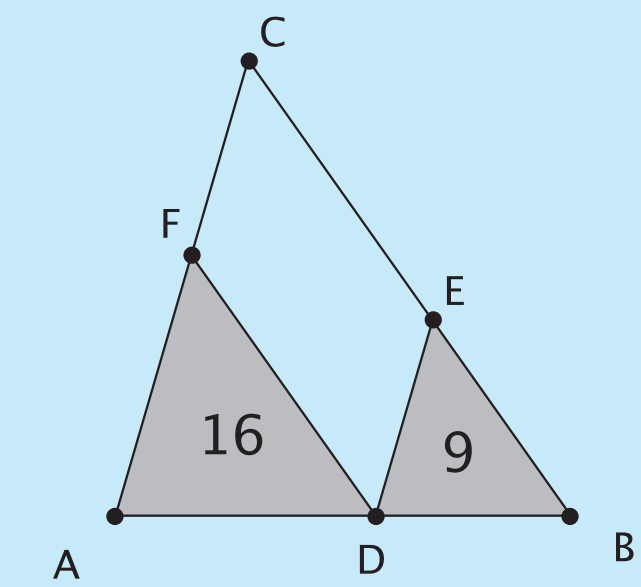# Geometry Problem

Geometry Level 3In image above the lines $DE$ and $DF$ are parallel to sides $AC$ and $BC$ of the triangle $ABC$ The triangles $ADF$ and $DBE$ are triangles with area $16$ and $9$ respectively. What is the area of $CFDE$?

×